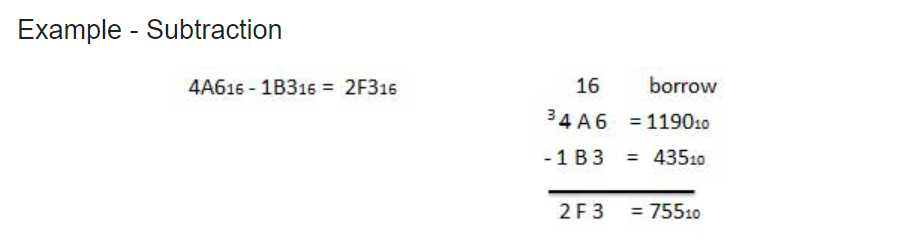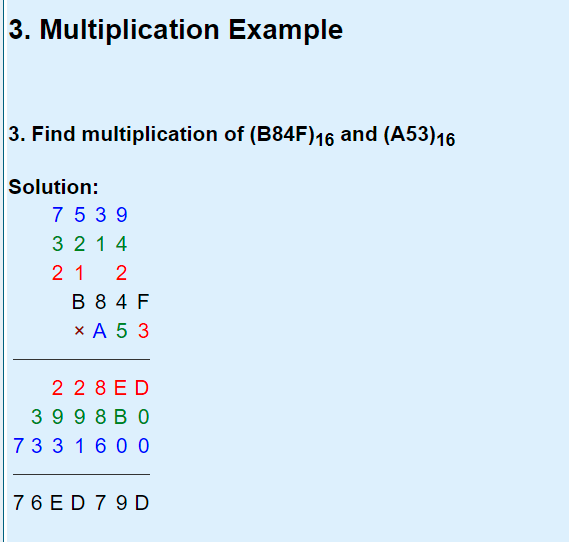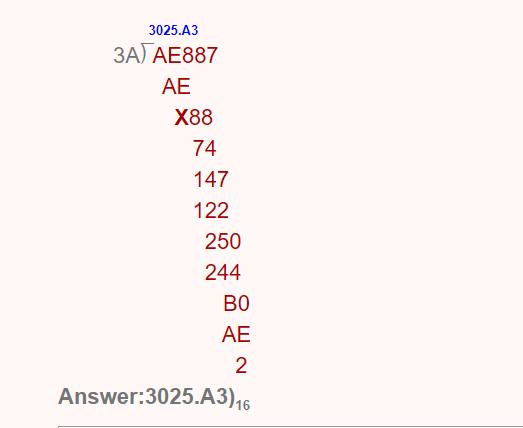To use prepostseo Hexadecimal Calculator, enter the values in the input boxes below and click on the Calculate button.

### What is Hex?

Hex or Hexadecimal number system works similarly to the binary and the decimal systems. The numbers 10 or 2 as a base are not considered, the number 16 as a base is used. Hexadecimal number system considers 16 digits consisting of the number 0 to 9. It also uses the alphabets A, B, C, D, E, and F to denote the numbers from 10 to 15.

Hexadecimal number system considers the same concept of the addition of the decimal system. However, the numbers being represented by the alphabetical letters A,B,C,D,E, and F. The addition system in Hex is based on computing the basic addition of decimal at the same time transforming from hex system to decimal system when the values greater than the number 9 is available. Hex addition example is given below:## Hex Subtraction

The system of subtraction in Hex is similar to the process of addition. But, when the borrowing is done in Hex “1” which is borrowed denotes 16decimal and not 10decimal. So, the column from which it has been taken is 16 times more than the column from which it has been borrowed. The conversions and computations of the alphabetical letters A, B, C, D, E, and F are performed properly, the subtraction process is not at all difficult when compared with the decimal subtraction. Hex subtraction example is given below:## Hex Multiplication

The multiplication performed in the Hex decimal system can be a bit twisty because when the computations are done from the hex system to the decimal system involve more attention and care because the numbers are of greater value. Hex multiplication example is given below:## How the division is done?

The greater calculations in the hex system are similar to the greater calculations of division in decimal. The users can compute to decimal and carry out greater calculations of division in decimal, then it can be computed back again from where it was started. Please, see an example of hex division below:## Hex Calculator

Hexadecimal calculator is used for hex addition, hex subtraction, hex multiplication, and hex division without pen and paper. Hex adder calculator is an effcient tool for adding two hex values. Similarly, hex calculator can easily calculate two given values into 4 different calculations i.e., multiplication, division, subtratction, and addition. Hex to binary and binary to hex converter can be used if you want to convert numbers to hex instead of calculating them.

HomeTools › Hex Calculator
— Languages —